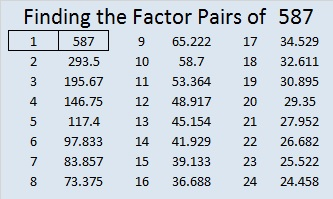# 587 and Level 2

587 is the sum of the five prime numbers from 107 to 131.Print the puzzles or type the solution on this excel file: 10 Factors 2015-08-17

—————————————————————————————————

• 587 is a prime number.
• Prime factorization: 587 is prime.
• The exponent of prime number 587 is 1. Adding 1 to that exponent we get (1 + 1) = 2. Therefore 587 has exactly 2 factors.
• Factors of 587: 1, 587
• Factor pairs: 587 = 1 x 587
• 587 has no square factors that allow its square root to be simplified. √587 ≈ 24.22808How do we know that 587 is a prime number? If 587 were not a prime number, then it would be divisible by at least one prime number less than or equal to √587 ≈ 24.2. Since 587 cannot be divided evenly by 2, 3, 5, 7, 11, 13, 17, 19, or 23, we know that 587 is a prime number.

—————————————————————————————————This site uses Akismet to reduce spam. Learn how your comment data is processed.